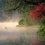# Geometry Again!

Following are some geometry questions I couldnot solve. They may be from other olympiads, and if their solutions are available online please point me to them.

1. For a pentagon $$ABCDE,\ AE=ED,\ AB+CD=BC,$$ and $$\angle BAE + \angle CDE=180 ^{\circ}$$. P.T. $$\angle AED=2\angle BEC$$.

2. Let P be a point inside a triangle with $\angle C=90 ^{\circ}$ such that $AP=AC$, and let M be the midpt of AB and CH be the altitude. Prove that PM bisects $\angle BPH$ iff $\angle A=60 ^{\circ}$.Note by Megh Parikh
7 years, 5 months ago

This discussion board is a place to discuss our Daily Challenges and the math and science related to those challenges. Explanations are more than just a solution — they should explain the steps and thinking strategies that you used to obtain the solution. Comments should further the discussion of math and science.

When posting on Brilliant:

• Use the emojis to react to an explanation, whether you're congratulating a job well done , or just really confused .
• Ask specific questions about the challenge or the steps in somebody's explanation. Well-posed questions can add a lot to the discussion, but posting "I don't understand!" doesn't help anyone.
• Try to contribute something new to the discussion, whether it is an extension, generalization or other idea related to the challenge.

MarkdownAppears as
*italics* or _italics_ italics
**bold** or __bold__ bold
- bulleted- list
• bulleted
• list
1. numbered2. list
1. numbered
2. list
Note: you must add a full line of space before and after lists for them to show up correctly
paragraph 1paragraph 2

paragraph 1

paragraph 2

[example link](https://brilliant.org)example link
> This is a quote
This is a quote
    # I indented these lines
# 4 spaces, and now they show
# up as a code block.

print "hello world"
# I indented these lines
# 4 spaces, and now they show
# up as a code block.

print "hello world"
MathAppears as
Remember to wrap math in $$ ... $$ or $ ... $ to ensure proper formatting.
2 \times 3 $2 \times 3$
2^{34} $2^{34}$
a_{i-1} $a_{i-1}$
\frac{2}{3} $\frac{2}{3}$
\sqrt{2} $\sqrt{2}$
\sum_{i=1}^3 $\sum_{i=1}^3$
\sin \theta $\sin \theta$
\boxed{123} $\boxed{123}$

Sort by:

For Problem 1. Consider the point $F$ in the ray $BA$ such that $AF=CD$ ($A$ between $B$ and $F$), then triangles $FAE$ and $CDE$ are congruent (using $AE=ED$ and $\angle BAE+\angle CDE=180^\circ$. Note that the triangles $CEB$ and $FEB$ are congruent because $EC=EF$ and $BC=AB+CD=AB+AF=BF$. Finally, $\angle BEC=\angle BEF=\angle BEA+\angle AEF=\angle BEA+\angle DEC$ therefore $\angle AED=2\angle BEC$.

- 7 years, 5 months ago

Thanks.

- 7 years, 5 months ago

This problem wouldn't be so hard once you notice a property that $P$ carries from $AC=AP$.

WLOG say that $H$ is between $A,M$. Since $AC=AH\times AB$, therefore $AP=AH*AB$ which is enough to show that $\triangle APH\sim \triangle ABP\Rightarrow \frac {PH}{PB}=\frac {AP}{AB}=\frac {AC}{AB}$. Since $PM$ bisects $\angle BPH\iff \frac {PH}{PB}=\frac {HM}{BM}$ combining the results above we continue the equivalence: $\iff \frac {AC}{AB}=\frac {HM}{BM}=\frac {2HM}{AB}$ $\iff AC=2HM$.

Instead to computing for $HM$, we can geometrically show that $AC=2HM\iff \angle A$. To relate $AC$ and $HM$, we introduce the midpoint of $AC$(I actually originally connected $GH$, but then I found a simpler way). Since $\angle AGM=\angle CHM=90$($CM=AM$) and $AG=\frac {AC}{2}=HM$, by $HL$ congruence we have $\angle GAM=\angle CMA=\angle ACM\Rightarrow \angle CAB=60$. Q.E.D

For the first one I have the same proof as Jorge :)

- 7 years, 5 months ago

As a crazy thought for this problem, we could also argue by considering when Apollonius' circle of $H,B;M$ and the circle centered at $A$ with radius $AC$ intersect.

- 7 years, 5 months ago

Thanks.

- 7 years, 5 months ago